Next: 3.2.3 The Relaxation Function Up: 3.2 Measuring the Internal Previous: 3.2.1 The Raw Asymmetry

## 3.2.2 The Corrected Asymmetry

In practice, there are differences in the efficiency of the counters and also geometrical misalignment of the counters. If the sensitivity of the individual positron counters is not the same, the normalization constant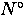, the maximum decay asymmetry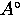and the random background levels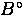will not be the same in all of the counters. The number of recorded events in each counter also depends on the solid angle they subtend at the sample. If the coverage of the solid angle is not maximized because of counter misalignments, there will be a decrease in the number of decay positrons detected. The raw asymmetry thus depends on the variables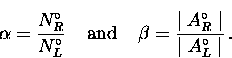(18)
In a superconductor, these variables are best determined by fitting the muon spin precession signal in the normal state where the magnetic field in the sample is more or less homogeneous. The corrected asymmetry''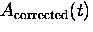is obtained by substituting Eq. (3.18) into Eq. (3.10) so that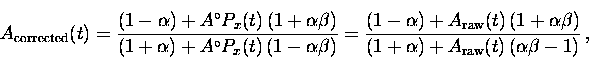(19)
where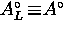and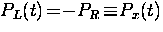.Next: 3.2.3 The Relaxation Function Up: 3.2 Measuring the Internal Previous: 3.2.1 The Raw Asymmetry